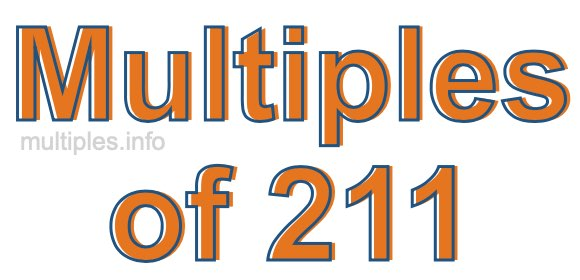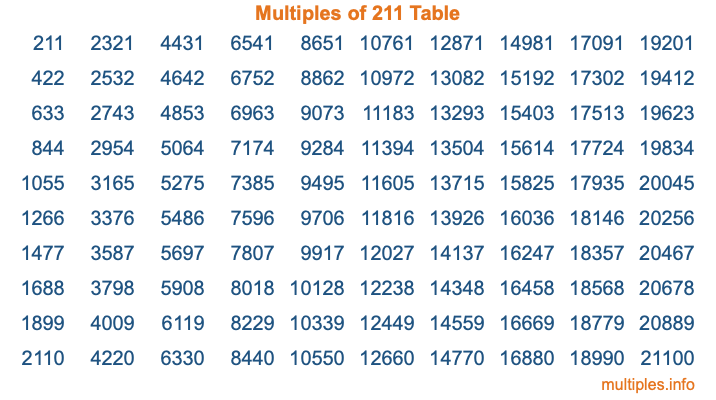Multiples of 211Welcome to the Multiples of 211 page. Here we will first teach you everything you will ever need to know about the multiples of 211, and then give you a study guide summary of everything we taught you to make sure you remember it all. Use this page to look up facts and learn information about the multiples of 211. This page will make you a multiples of two hundred eleven expert!

Definition of Multiples of 211
Multiples of 211 are all the numbers that when divided by 211 equal an integer. Each of the multiples of 211 are called a multiple. A multiple of 211 is created by multiplying 211 by an integer.

Therefore, to create a list of multiples of 211, you start with 1 multiplied by 211, then 2 multiplied by 211, then 3 multiplied by 211, and so on for as long as you want. Thus, the list of the first five multiples of 211 is 211, 422, 633, 844, and 1055. To see a larger list of multiples of 211, see the printable image of Multiples of 211 further down on this page. We also have a category where you can choose any nth multiple of 211.

Multiples of 211 Checker
The Multiples of 211 Checker below checks to see if any number of your choice is a multiple of 211. In other words, it checks to see if there is any number (integer) that when multiplied by 211 will equal your number. To do that, we divide your number by 211. If the the quotient is an integer, then your number is a multiple of 211.

Is  a multiple of 211?

Least Common Multiple of 211 and ...
A Least Common Multiple (LCM) is the lowest multiple that two or more numbers have in common. This is also called the smallest common multiple or lowest common multiple and is useful to know when you are adding our subtracting fractions. Enter one or more numbers below (211 is already entered) to find the LCM.

Check out our LCM Calculator if you need more details about the Least Common Multiple or if you need the LCM for different numbers for adding and subtraction fractions.

nth Multiple of 211
As we stated above, 211 is the first multiple of 211, 422 is the second multiple of 211, 633 is the third multiple of 211, and so on. Enter a number below to find the nth multiple of 211.

th multiple of 211

Multiples of 211 vs Factors of 211
211 is a multiple of 211 and a factor of 211, but that is where the similarities end. All postive multiples of 211 are 211 or greater than 211. All positive factors of 211 are 211 or less than 211.

Below is the beginning list of multiples of 211 and the factors of 211 so you can compare:

Multiples of 211: 211, 422, 633, 844, 1055, etc.

Factors of 211: 1, 211

As you can see, the multiples of 211 are all the numbers that you can divide by 211 to get a whole number. The factors of 211, on the other hand, are all the whole numbers that you can multiply by another whole number to get 211.

It's also interesting to note that if a number (x) is a factor of 211, then 211 will also be a multiple of that number (x).

Multiples of 211 vs Divisors of 211
The divisors of 211 are all the integers that 211 can be divided by evenly. Below is a list of the divisors of 211.

Divisors of 211: 1, 211

The interesting thing to note here is that if you take any multiple of 211 and divide it by a divisor of 211, you will see that the quotient is an integer.

Multiples of 211 Table
Below is an image of the first 100 multiples of 211 in a table. The table is in chronological order, column by column. The first column has the first ten multiples of 211, the second column has the next ten multiples of 211, and so on.The Multiples of 211 Table is also referred to as the 211 Times Table or Times Table of 211. You are welcome to print out our table for your studies.

Negative Multiples of 211
Although not often discussed or needed in math, it is worth mentioning that you can make a list of negative multiples of 211 by multiplying 211 by -1, then by -2, then by -3, and so on, to get the following list of negative multiples of 211:

-211, -422, -633, -844, -1055, etc.

Multiples of 211 Summary
Below is a summary of important Multiples of 211 facts that we have discussed on this page. To retain the knowledge on this page, we recommend that you read through the summary and explain to yourself or a study partner why they hold true.

There are an infinite number of multiples of 211.

A multiple of 211 divided by 211 will equal a whole number.

211 divided by a factor of 211 equals a divisor of 211.

The nth multiple of 211 is n times 211.

The largest factor of 211 is equal to the first positive multiple of 211.

211 is a multiple of every factor of 211.

211 is a multiple of 211.

A multiple of 211 divided by a divisor of 211 equals an integer.

211 divided by a divisor of 211 equals a factor of 211.

Any integer times 211 will equal a multiple of 211.

Multiples of a Number
Here you can get the multiples of another number, all with the same attention to detail as we did for multiples of 211 on this page.

Multiples of
Multiples of 212
Did you find our page about multiples of two hundred eleven educational? Do you want more knowledge? Check out the multiples of the next number on our list!